# BODMAS RULE | PEDMAS Rule | MATH Solutions

BODMAS is the abbreviation of Bracket, Division, Multiplication, Addition and Subtraction. In some countries this rules is defined as PEDMAS means Parentheses, Exponents, Division, Multiplications, Additions and Subtraction. This is step by step order of solving Mathematics expressions.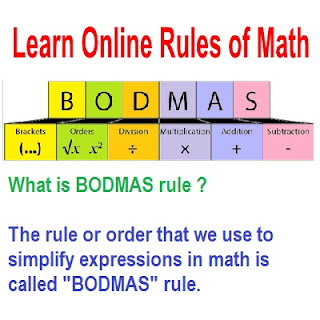Learn Online Rules BODMAS

Today here you will learn about "BODMAS Rules" Mathematics for the all class Students. BODMAS is a Rule in which by using BODMAS rule or BODMS Order anyone can easily solve mathematics expressions so it is called "BODMAS" Rule.

Complete Meanings of BODMAS explained below here:

B = Brackets Solved First
O = OF (Orders-> Powers And Radicals)
D = Division
M = Multiplications
A = Additions
S = Subtractions

Some Important Tips For Solving Questions BODMAS Rule:

Rule 1. In a particular simplifications if you have both multiplications and divisions, do the operations one by one in the order from left to right.

Rule 2. Division does not always come before multiplication. We have to do one by one in the order from left to right.

Rule 3. In a particular simplification, if you have both addition and subtraction, do the operations one by one in the order from left to right.BODMAS RULE Math Example 1BODMAS RULE Math Example 2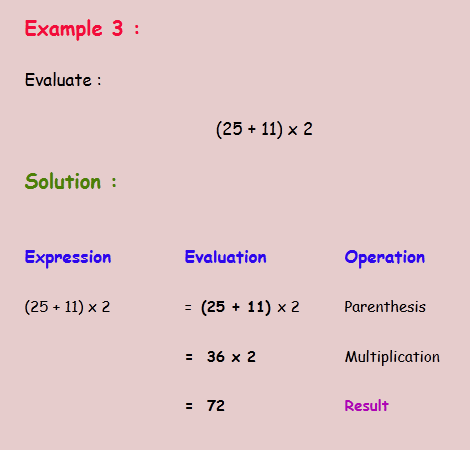BODMAS RULE Math Example 3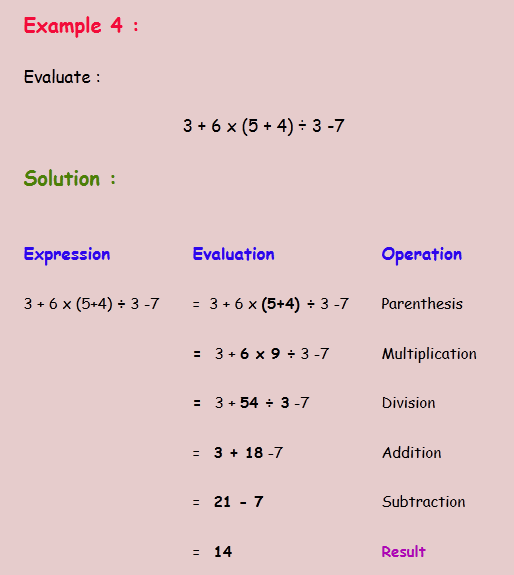BODMAS RULE Math Example 4BODMAS RULE Math Example 5BODMAS RULE Math Example 6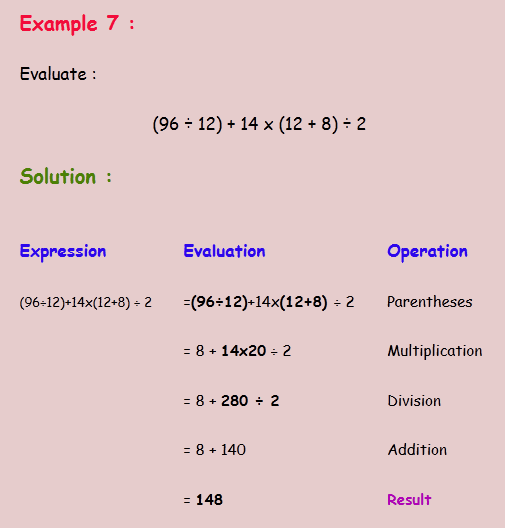BODMAS RULE Math Example 7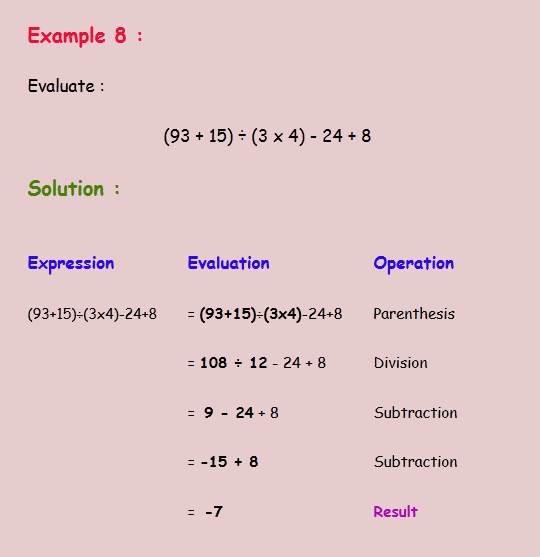BODMAS RULE Math Example 8BODMAS RULE Math Example 9BODMAS RULE Math Example 10

All of these given examples help the students and teachers for learning math important rules of BODMAS Math expressions. Solved step by step mathematics rules are explained in these simple questions with solutions.

Previous Post
Next Post